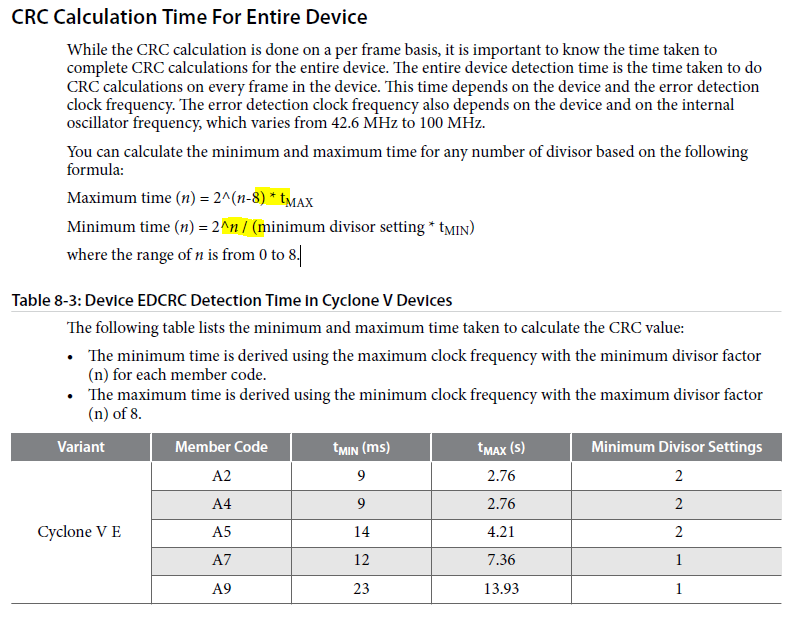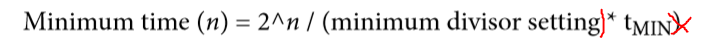Programmable Devices
CPLDs, FPGAs, SoC FPGAs, Configuration, and Transceivers
Announcements
19988 Discussions

## CRC in Cyclone V DevicesBeginner
690 Views

Hi,

I have got questions regarding the CRC capability of Cyclone V devices:

The following document describes the behaviour on pages 291 - 301:

https://www.intel.com/content/dam/www/programmable/us/en/pdfs/literature/hb/cyclone-v/cv_5v2.pdf

The Document gives the following description:

"While the CRC calculation is done on a per frame basis, it is important to know the time taken tocomplete CRC calculations for the entire device. The entire device detection time is the time taken to doCRC calculations on every frame in the device. This time depends on the device and the error detectionclock frequency. The error detection clock frequency also depends on the device and on the internaloscillator frequency, which varies from 42.6 MHz to 100 MHz."

Now my questions:

1) What is the meaning of a "frame"? Does a Cyclone V device has one frame, or more? If more, where can I get the number of frames?

2) It is described, that the error detection frequency (internal oszillator frequency) varies from 42.6 MHz to 100 MHz. Where is the error detection frequency of a device defined? Where can I get this information?

My goal is to calculate the time to calculate a CRC detection over the whole FPGA in user mode.

Best regards,

David

8 RepliesBeginner
335 Views

I attached an extract of the document.

For me also the formulas are still not clear. I highlighted a part in yellow. So, the maximum time is calculated by a division, the minimum time with a multiplication. This can't work due to units. One time, we devide by seconds, the other we multiply with seconds and the result shall be a number with the unit seconds. So, where is the failure?

Again, I'm interested in the time the Cyclone V needs for a CRC detection over the whole device...

Thanks!Employee
336 Views

HI,

1) What is the meaning of a "frame"? Does a Cyclone V device has one frame, or more? If more, where can I get the number of frames?

Each device will have a certain number of frame. We do not release the frame number but it will be within 16bit addressing for Cyclone V

2) It is described, that the error detection frequency (internal oszillator frequency) varies from 42.6 MHz to 100 MHz. Where is the error detection frequency of a device defined? Where can I get this information?

It is define in the Quartus setting.

If you look into the time used, Tmin using ms while Tmax is in second. That is the reason of using different calculation.Beginner
336 Views

Hi JohnT,

"It is define in the Quartus Setting."

--> Where is it defined?+

If you look into the time used, Tmin using ms while Tmax is in second. That is the reason of using different calculation.

--> But one time, I have a devision and one time, I have a multiplication. This can't be correct due to the units. If I devide by [s] it is not the same than multiply with [s], if I expect an result in [s].

--> Can you please clarify or give me an example, e.g. for the Cyclone V E A9. Therefore Tmin = 23ms and Tmax is 13.93s. So, how to calculate the minimum and maximum time for CRC of the whole device?

Thanks and best regards,

DavidEmployee
336 Views

Hi David,

After checking with engineering below is the answer.

--> Where is it defined?

Setting can be change as the oscillator is fixed. The frequency will varies from device to device and it will be between from 42.6Mhz to 100Mhz.

--> Can you please clarify or give me an example, e.g. for the Cyclone V E A9. Therefore Tmin = 23ms and Tmax is 13.93s. So, how to calculate the minimum and maximum time for CRC of the whole device?

There is error on the calculation of the minimum time where the bracket should be there.

The Table 106 is showing the time for full device CRC calculation.

So the Tmax is calcluated based on the maximum divisor factor of n = 8. So 13.93s = 2^(8-8)*13.93s.

The Tmin is calculated based on the minimum divisor factor based on the device minimum divisor setting supported. For Cyclone V E A9 then it will be 23s = 2^0 / 1 * 23. The value Divisor Setting is 2 ^ n. So n = 0 when the divisor setting is 1 ( 2^0 = 1).

Thanks.

John TioBeginner
336 Views

Hi John,

Setting can be change as the oscillator is fixed. The frequency will varies from device to device and it will be between from 42.6Mhz to 100Mhz.

--> I think, you mean "Setting cannot be change as the oscillator is fixed.", right?

There is error on the calculation of the minimum time where the bracket should be there.

--> So, I assume, the right formula is as shown above, can you confirm?The Table 106 is showing the time for full device CRC calculation.

--> What do you mean with "Table 106"? I guess, you are talking about "Table 8-3: Device EDCRC Detection Time in Cyclone V Devices". Can you confirm?

--> So, I understand, that this table already shows the minimum and maximum detection time for CRC errors in the whole device and I don't have to calculate those numbers. Did I get this right?

So the Tmax is calcluated based on the maximum divisor factor of n = 8. So 13.93s = 2^(8-8)*13.93s.

--> Ok.

The Tmin is calculated based on the minimum divisor factor based on the device minimum divisor setting supported. For Cyclone V E A9 then it will be 23s = 2^0 / 1 * 23. The value Divisor Setting is 2 ^ n. So n = 0 when the divisor setting is 1 ( 2^0 = 1).

--> The result is 23ms and not 23s, right? I have to be very sure, that I get this right...

Thanks and best Regards,

DavidEmployee
336 Views

Hi David,

--> I think, you mean "Setting cannot be change as the oscillator is fixed.", right?

Yes, you are correct.

There is error on the calculation of the minimum time where the bracket should be there.

--> So, I assume, the right formula is as shown above, can you confirm?Yes, I already feedback this issue to be fixed in next released.

The Table 106 is showing the time for full device CRC calculation.

--> What do you mean with "Table 106"? I guess, you are talking about "Table 8-3: Device EDCRC Detection Time in Cyclone V Devices". Can you confirm?

--> So, I understand, that this table already shows the minimum and maximum detection time for CRC errors in the whole device and I don't have to calculate those numbers. Did I get this right?

Yes. but if you need specific divider used in your design then you can calculate what is the maximum and minimum time needed.

So the Tmax is calcluated based on the maximum divisor factor of n = 8. So 13.93s = 2^(8-8)*13.93s.

--> Ok.

The Tmin is calculated based on the minimum divisor factor based on the device minimum divisor setting supported. For Cyclone V E A9 then it will be 23s = 2^0 / 1 * 23. The value Divisor Setting is 2 ^ n. So n = 0 when the divisor setting is 1 ( 2^0 = 1).

--> The result is 23ms and not 23s, right? I have to be very sure, that I get this right...

Yes you are correct. Sorry for the conffussion.Beginner
336 Views

​Hi John,

Thanks for clarification!

Best regards,

DavidEmployee
336 Views

Hi David,

The documentation has also been updated to the correct calculation.